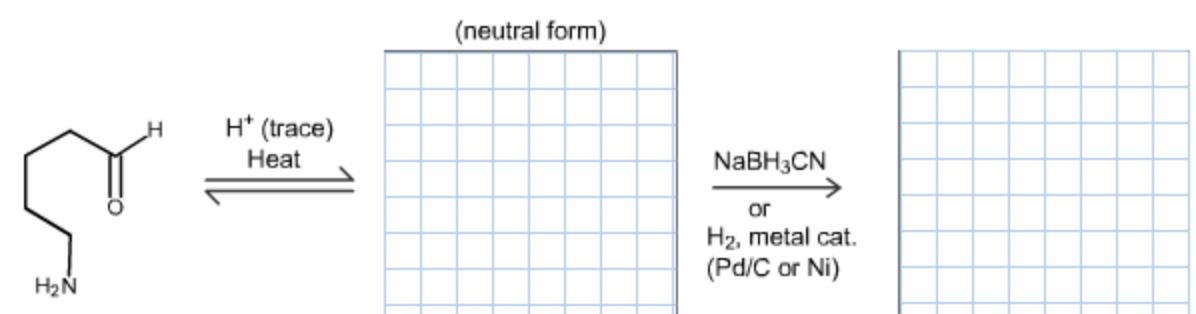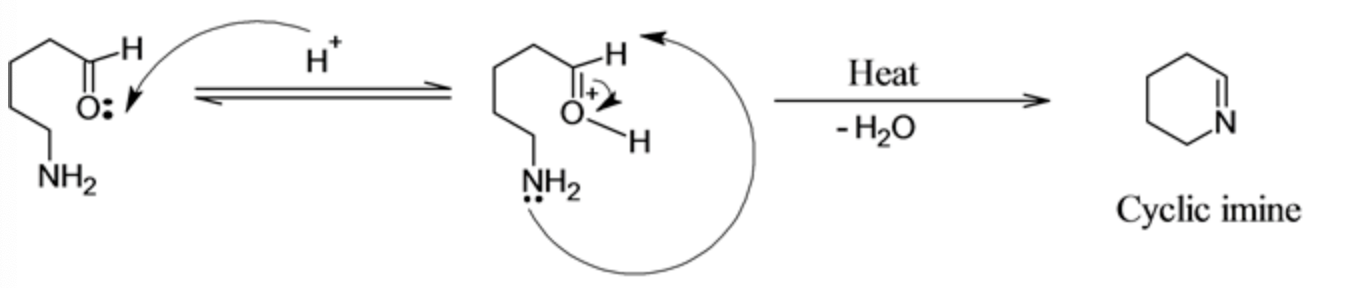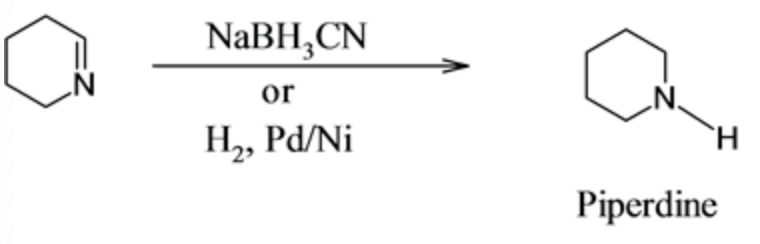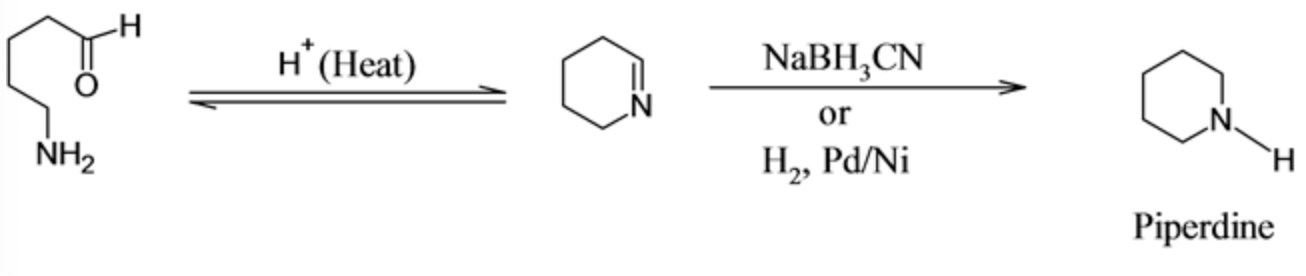In: Chemistry

# Draw the structure of the organic product of each reaction in the following two-step synthesis.

Draw the structure of the organic product of each reaction in the following two-step synthesis.## Solutions

##### Expert Solution

Concepts and reason

The concept used to solve the given problem is the knowledge of the nucleophilic addition reaction on the carbonyl carbon.

Fundamentals

The carbonyl carbon has the electrophilic carbon which is deficient in electrons and is fulfilled by the nucleophilic attack which results in the nucleophilic addition product. The nucleophile attacks the $$\pi^{*}$$ antibonding orbital of the carbonyl carbon result in the breakage of the $$\pi$$ bond between $$C$$ and $$O$$.

In the given reactant the proton attacks the lone pair of oxygen which further proceed with the intramolecular attack of $$\mathrm{NH}_{2}$$ as a nucleophile on the carbonyl results in the formation of cyclic imine. The mechanism of this reaction is given below:The proton attacks on the lone pair of oxygen creates the positive charge on oxygen, this increases the electrophilicity of carbonyl carbon. Now the internal amino group as a nucleophile attack on the carbonyl carbon, results in the formation of cyclic imine and water leaves as the leaving group.

When the cyclic imine reacts with reducing agent such as $$\mathrm{NaBH}_{3} \mathrm{CNorH}_{2} / \mathrm{PdorNi}$$ undergoes reduction and the imine group converts into the cyclic secondary amine which is commonly known as piperdine. The reaction of reduction of cyclic imine is as follows:The given reagents $$\mathrm{NaBH}_{3} \mathrm{CNorH}_{2} / \mathrm{PdorNi}$$ selectively reduces the cyclic imine group into secondary amine. It does not break the ring to form the primary amine.

The structure of the organic product of each reaction for the two-step synthesis are as follows: